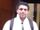## Summary statistics

Hello,

I am checking someone else's results, basic summary. We got the same N counts, same Means but different standard deviations. What could be the reason?

Thanks!

9 REPLIES 9novinosrin
Tourmaline | Level 20

## Re: Summary statistics

"We got the same "  do you mean are you comparing yours with his/her's

if yes, the datapoints seem to vary or spread differently between yours and someone else's

for eg:

``````data w;
k=mean(4,4,4);
std_k=std(4,4,4);
k1=mean(2,4,6);
std_k1=std(2,4,6);
run;``````

## Re: Summary statistics

we supposed to have used the same dataset. In any cases, that could result in same Ns, means but different standard deviations. But since we are checking independently, his/her dataset could have changed a little, such as slight updates for some values.novinosrin
Tourmaline | Level 20

## Re: Summary statistics

@TX_STAR wrote:
we supposed to have used the same dataset. In any cases, that could result in same Ns, means but different standard deviations. But since we are checking independently, his/her dataset could have changed a little, such as slight updates for some values.

With N< 30 and the possibility of data having changed you're likely not actually using the same data. And thus the expectation of the same variance is not correct.

## Re: Summary statistics

@TX_STAR wrote:

Hello,

I am checking someone else's results, basic summary. We got the same N counts, same Means but different standard deviations. What could be the reason?

Thanks!

How different? Are you talking 6th decimal places or it's off by a lot?

## Re: Summary statistics

first decimal. N <30

## Re: Summary statistics

Which procedure are you using?

Exact same data set or did you build a different set?

Maybe show the code you used and some output for your code and your coworker's output (if you don't have the code).

If the difference is very small it could be hardware differences such as different chipset. If running a different OS then that would be another potential for small differences. And another could just be setting formats or numbers of decimals for display.

## Re: Summary statistics

Do a Proc Compare, it would be handy in zeroing down on the exact observations and the values that are varying between the two data sets.

## Re: Summary statistics

Hello

Thank you all for your help. I finally found out what caused the same mean but different standard deviation using the same data set. In proc mean I used the default, the other person used additional option VARDEF=n. So different SD formulas were applied when calculating the SD, SD for a sample (divided by n-1) and SD for population (divided by n).

Thanks!

Discussion stats
• 9 replies
• 710 views
• 2 likes
• 5 in conversation